# Civil Engineering - Steel Structure Design

### Exercise :: Steel Structure Design - Section 3

36.

A single angle in tension is connected by one leg only. If the areas of connecting and outstanding legs are respectively a and b, net effective area of the angle, is

 A.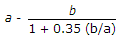B.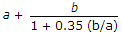C.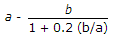D.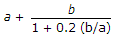E. none of these.

Explanation:

No answer description available for this question. Let us discuss.

37.

The central deflection of a simply supported steel beam of length L with a concentrated load W at the centre, is

 A.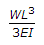B.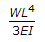C.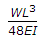D.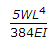Explanation:

No answer description available for this question. Let us discuss.

38.

If L is the overall length of a combined footing having A as its area, d being the distance between the centre of gravity of the base and centre of the base, the larger width b is

 A.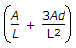B.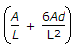C.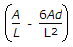D.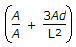Explanation:

No answer description available for this question. Let us discuss.

39.

On eccentrically loaded columns, the equivalent axial load may be obtained by

 A. adding the axial load, eccentric load, the product of the bending moment due to eccentric load and the appropriate bending factor B. adding the axial load and eccentric load and subtracting the product of bending moment and appropriate bending factor C. dividing the sum of axial load and eccentric load by the product of the bending moment and appropirate bending factor D. none of these.

Explanation:

No answer description available for this question. Let us discuss.

40.

The distance between the outer faces of flanges of a plate girder, is known as

 A. overall depth B. clear depth C. effective depth D. none of these.